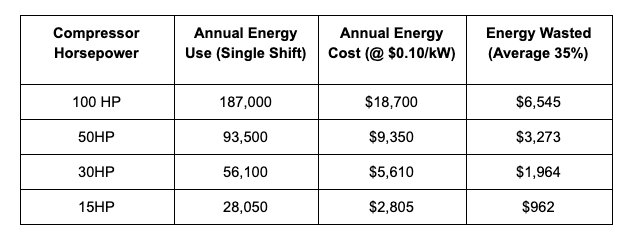# Compressed Air Leak Calculator

## Compressed air leaks are costing you money

Leaks in your compressed air system may be increasing your compressed air energy bills by more than 30%. Since compressed air is usually one of the largest energy expenses for a manufacturer using air-powered equipment, that represents a significant opportunity for energy savings. Compressed air leaks are widespread in all applications. Not only are they common, but they are time-consuming to find and fix. In the average manufacturing operation, 20-30% of the total energy consumption is compressed air. On average, 30% of that expense is due to compressed air leakage.

A general guideline when calculating the cost of a compressed air leak is that one CFM* of air costs about \$35 per shift annually (assuming an average energy cost of about \$0.08/kW). If you run multiple shifts, multiply that number by 2 or 3; if your plant runs 24/7/365, multiply it by 4.2. For the average manufacturer, 20-30% of total energy consumption is compressed air; 35% of that energy is wasted due to air leaks and other inefficiencies. Read more on how much air leaks can be costing your facility.

## How to calculate cost of air leaks

The air leakage calculation formula lets you determine how much energy you are wasting per year. To calculate the cost of leaks in your systems, begin by multiplying the compressor horsepower by kW conversion rate of .746 to determine the kW used. Next use your energy cost (you can use \$0.12 if unknown) and multiply that by the kW used to come up with your hourly energy cost. Now take the hourly energy cost and multiply that by your leak rate (if unknown use the average leak rate of .35). This will give you how much leaks are costing you per hour. Finally use your average operating hours and multiply that by the per hour cost and we have what your air leaks are costing you per year.

## Compressed air leakage chart

By looking at the chart below you can see how much energy dollars are being wasted when leaks and inefficiencies are not repaired.Chicago (847) 999-7474

Minneapolis (612) 445-3184

Milwaukee (414) 895-5338

Detroit (248) 775-1459